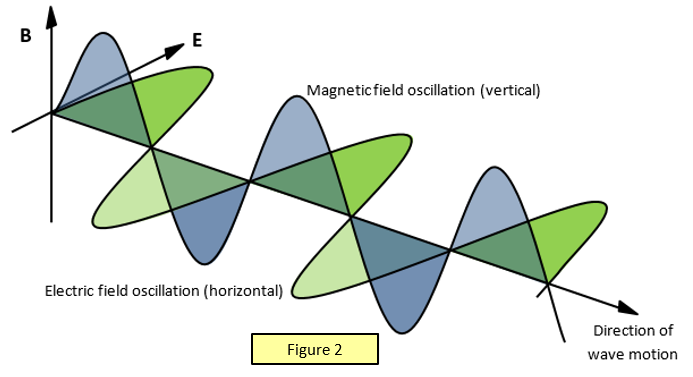# Why is light a transverse wave?

Apr 28, 2015

Light is a transverse wave because its components vibrate perpendicular to the direction of propagation.

A transverse wave is a wave that oscillates perpendicular to its direction of propagation.

A light wave is an electromagnetic wave.

It has an electric and a magnetic component.As the wave propagates in (say) the $z$ direction, the electric field is oscillating in (say) the $y$ direction and the magnetic field is oscillating in the $x$ direction.

Since the $x$ and $y$ axes are perpendicular to the $z$ axis, light is a transverse wave.